#### Termination

The sampling-based planning algorithms in Chapter 5 were designed to terminate upon finding a solution path. In the current setting, termination is complicated by the fact that we are interested in solutions from all initial configurations. Since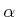is dense, the volume of uncovered points intends to zero. After some finite number of iterations, it would be nice to measure the quality of the approximation and then terminate when the desired quality is achieved. This was also possible with the visibility sampling-based roadmap in Section 5.6.2. Using random samples, an estimate of the fraction ofcan be obtained by recording the percentage of failures in obtaining a sample inthat is outside of the cover. For example, if a new neighborhood is created only once in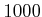iterations, then it can be estimated that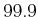percent ofis covered. High-probability bounds can also be determined. Termination conditions are given in  that ensure with probability greater than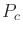that at least a fraction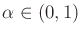ofhas been covered. The constantsandare given as parameters to the algorithm, and it will terminate when the condition has been satisfied using rigorous statistical tests. If deterministic sampling is used, then termination can be made to occur based on the dispersion, which indicates the largest ball inthat does not contain the center of another neighborhood. One problem with volume-based criteria, such as those suggested here, is that there is no way to ensure that the cover preserves the connectivity of. If two portions ofare connected by a narrow passage, the cover may miss a neighborhood that has very small volume yet is needed to connect the two portions.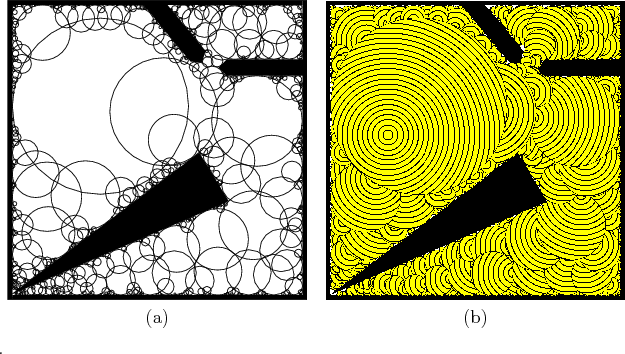Example 8..18 (2D Example of Computed Funnels)   Figure 8.18 shows a 2D example that was computed using random samples and the algorithm in Figure 8.17. Note that once a cover is computed, it can be used to rapidly compute different navigation functions and vector fields for various goals. This example is mainly for illustrative purposes. For the case of a polygonal environment, constructing covers based on convex polygons would be more efficient.Steven M LaValle 2020-08-14